• 42
•
• 3
•
• 4
•
•
•
49
Shares

# The motion of the charged particles in the combined electric and magnetic field

We have discussed the motion of charged particles in a uniform magnetic field and electric field separately. We have seen that what is the behaviour of the charged particles in the magnetic fields and electric fields. In this present article, we will discuss the combined motion of the charged particles in the uniform electric and magnetic fields. So let’s get started…

## Introduction

Whenever a charged particle moves in the simultaneous presence of both electric and magnetic fields then the particle has a variety of manifestations related to its motion. Its motion can be ranged from straight-line motion to cycloid or even very complex motion.

As we know that both electric and magnetic fields can impart acceleration to the charged particle. But, the magnetic field’s speciality is that the acceleration due to the magnetic field relates only to the change of direction of motion. But acceleration due to electric field can change both directions as well as magnitude.

If a charged particle is moving then the magnetic force being always perpendicular to the velocity of the particle and it tends to move the particle along a circular path. But on the other hand, the electric force acts along the electric field and it is capable to bring changes in both direction and magnitude depending upon the initial velocity and direction of the charged particle with respect to the electric field. If velocity is perpendicular to the electric vectors, then the particle follows a parabolic path.

There are various alignments of electric field and the magnetic field but one of the important alignments of electric and magnetic fields is termed as “crossed fields”. Here in the whole article, we use the term “crossed fields” to demonstrate the simultaneous presence of electric and magnetic fields at the right angle.

The charged particles such as electrons show different types of behaviour under the crossed fields. Their behaviours are very relevant in the study of electromagnetic measurement and application like calculation of specific charge of the electron, cyclotron radius, cyclotron frequency etc.

Before we proceed, it is necessary that we understood that elementary charged particles. Elementary charged particles have the mass of the order of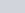kg or less. Therefore, due to their small masses, small electric or magnetic force are sufficient to generate very high acceleration of the order ofm/s² or more. Under proper setup, these particles can achieve velocity comparable to the speed of light.

To keep our discussion simple and non-boring, we will try to deal with the case which is less complicated and we will neglect the relativistic effects.

Some applications and phenomena linked with the simultaneous presence of the electric field of the magnetic field are given below:

• The motion of charged particles in uniform electric and magnetic fields.
• Calculation of specific charge of an electron (J.J Thomson experiment)
• Working of cyclotron

We will discuss cyclotron in a different article.

### The motion of a charged particle in simultaneous electric and magnetic field

We have already discussed the motion of the charged particles in uniform electric and magnetic fields through the different articles.

Check these articles

Here, we will combine the effects of both fields. Let’s discuss some of the interesting cases –

#### If a charged particle is moving parallel along electric and magnetic field

If charged particles are moving parallel along the electric field and magnetic field then the velocity, electric and magnetic field vectors will be in the same direction. Assume they are all aligned along the x-axis.

Since everything is aligned parallelly then it means magnetic field and velocity vectors will also parallel, if it does, then there is no magnetic force exist because the angle between the velocity vector and magnetic field vectors is 0°.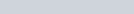where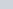is the initial velocity of the charged particle. During the motion, the charged particles can be accelerated or decelerated depending on the polarity of charges and the direction of the electric field. If the motion of the charged particle is in the same direction as the electric field then it will accelerate but if it is moving opposite of the electric field then it will decelerate.
Let’s Consider a positive charge, the electric force on the charged particle is given as: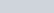The acceleration of the charged particle along x-direction is: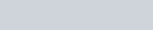From the second equation of motion, the displacement along x-axis after time t is given by:#### If a charged particle is moving perpendicular to the parallel electric and magnetic fields

Let’s assume that the electric and magnetic field vectors are aligned along the y-direction and the velocity vector is aligned along the positive x-direction.

Let’s take a positive charge whose initial velocity is. In this case, you see that the velocity and magnetic field vectors are perpendicular to each other. When we apply the right-hand rule to find the direction of magnetic force, then we find that the magnetic force is acting in the positive z-direction. If there is no electric field present, then the particle will revolve along a circular path in the xz plane as shown in the figure below.fig. 1, the motion of the charged particle in the electric and magnetic field, source: cnx.org

But here, the electric field is present along the y-direction. Thus, it will impart an acceleration in that direction. The particle, therefore, acquires velocity in the y-direction and the resulting motion will be a helical motion. But since the particle is accelerating in the y-direction, so the linear distance between the two consecutive circular loops of helix increases. In other words, the resulting motion will be a helical motion with increasing pitch.

The radius of each of the circular orbit and other related terms like time period, frequency and angular frequency for the case of the circular motion of the charged particle is perpendicular to the magnetic field is given as-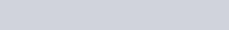##### The velocity of the charged particle

As we know that when there is no electric field then the charged particle revolves around a circular path in the xz plane. If it is revolving then it must have some velocity. The velocity of the charged particle revolving in the xz plane is given as-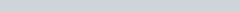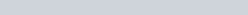Where α is the specific charge. We know that magnetic force couldn’t change the magnitude of the velocity of the charged particle. But, there is an electric field along the y-direction. This electric field imparts linear acceleration to the charged particle along the y-direction. Initially, the particle has no velocity component in y-direction but it gains velocity with time as the electric field imparts acceleration to the particle in the y-direction. But, the velocity components in the xz plane will remain the same. The acceleration of the charged particle in y-direction due to electric field is given as-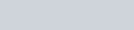Since the initial velocity of the charged particle along the y-direction is zero because its velocity vector is
aligned along the x-direction. But the velocity after time t is: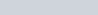The above equation is the velocity of the charged particle along the y-direction. Its velocity can be expressed vectorially in three-dimensionally as below: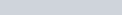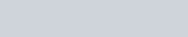##### Displacement of the charged particlefig. 3, displacement of the charged particle in the xz plane, source: cnx.org

Displacement of the charged particle in xz plane is given as-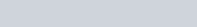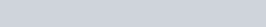The motion of the charged particle in the y-direction is due to the electric force. So let the displacement along y-direction be y after time t, then-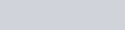After this motion, the position vector of the charged particle is-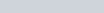Thus, it implies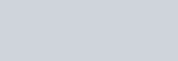#### If a charged particle is placed at rest in a crossed electric and magnetic field

If a charged particle is placed at rest in cross-field then it follows a cycloid path. Let’s see how? Here, we will skip the long complicated mathematical derivation and limit ourselves to the descriptive analysis only. We will discuss cycloid motion in detail in some other articles.

Consider the electric and magnetic fields which are directed along z and x directions. The charged particle is placed at the origin of the coordinate system. Since the charged particle is at rest, so there is no magnetic force acting on the particle. But, it is the electric force, which accelerates the charged particle in the z-direction.

As the particle acquires velocity in the z-direction, then the magnetic force comes into action and tries to rotate the particle in the xz plane about a centre on the x-axis.

For this rotation, magnetic force tries to draw the charged particle away from the z-axis along a curved path.

But, the z-component of the velocity keeps increasing with time due to electric force in that direction. The magnetic force constantly tries to draw the charged particle away from the z-axis along a curved path.

When magnetic force tries to draw the charged particle away from the z-axis then this action of magnetic force is countered by the electric force in the z-direction. The velocity of the charged particle finally comes to zero at the x-axis. This cycle repeats itself again and again and constitutes a cycloid motion.

You can imagine a cycloid motion by the motion of a point on the circumference of a rolling wheel. The path traced by the point during the motion of the wheel is cycloid. See figure below:

### Calculation of specific charge of an electron (J.J Thomson’s experiment)

The ratio of charge and mass of an electron is called the specific charge of an electron. The electron’s specific charge (α) is measured by introducing the crossed fields on a beam of electrons.

The beam of electrons emerges from the cathode plate (negatively charged) and passes through a very narrow hole in the centre of the anode plate (positively charged). This charge is acquired by the plates when it is connected to a high voltage source. When beams of electrons move from cathode to anode, they accelerated between the cathode and anode due to applied electrical potential V. The kinetic energy of the electron during the motion is given as-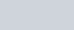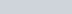Where v is the velocity of the electrons.
When electrons pass through the narrow hole of the anode, it enters into the cross electric and a magnetic field region. After passing this cross-field, electrons strike somewhere on the fluorescent screen with a glow. Let’s see how these cross fields are set up?
For deploying a cross-field, let’s first deploy the electric field, for this two parallel charged plates (positive plates lie at the upper side and negative plates at the lower side) are inserted along the z-axis into the glass container of the experimental setup.fig. 6, a labelled diagram of Thomson’s experiment, source: chemistry god

An electric field (E) produces from the positive plate to negative plates downward, when the electrons (negatively charged particle) tries to cross this field then it experiences an upwards electric force which acts in the opposite direction of the electric field because of the charge is negative. In the influence of electric force, electrons deviated upwards by taking a curve.

For deploying the magnetic field, a solenoid is used along the y-axis such that its north pole lies in the negative y-axis and the south pole lies in the positive y-axis covering the electric field plates. Here, the electric and magnetic field is perpendicular to each other i.e electric field is along the z-axis and magnetic field along the y-axis and the velocity of the particle is in the x-axis.

When electrons enter in the simultaneous region of cross-field then the electric force acted on the electrons is in an upwards direction but from the right-hand rule, magnetic force acting on the particle is in a downward direction.

We adjust the magnetic field until the electric force and magnetic force balance each other or the deviation of the electrons become zero. When the electrons attend zero deviation and start striking the fluorescent screen straightly along the x-axis, then in this case the magnetic force and electric force became equal and can be written as below: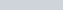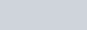Substitute this expression of v in the kinetic energy equation obtained earlier, then we have-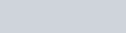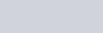As you can see that all the quantities on the right-hand side of the equation are measurable because everything is known to us. This equation allows us to measure the specific charge of electrons.

In fact, the calculation of specific charge of particles composing a cathode ray tube by J.J.Thomson is considered as the discovery of electrons. From this, it can also be easily inferred that he could determine the nature of the charge of an electron by studying the direction of deflection (upward or downward) when only either of the fields is in action.

In the above derivation, we measure potential difference which is used to accelerate the charged particle between cathode and anode. But we find that we can use some other quantities as well to determine the specific charges. We can measure the deflection of an electron beam when any field is active and use the data to determine the specific charge of an electron. Let’s investigate how can we use this data to find the specific charge of an electron.

#### Measurement of deflection by the magnetic field

When the magnetic and electric forces got balanced, then switch off the supply of the electric field and let the electron beam to be deviated due to the magnetic field. As we know that the magnetic force acts always perpendicular to the direction of motion.
Therefore, the particles move along a circular path inside the region of the magnetic field. When an electron passes out of the magnetic field, then it moves along the straight line and strikes the fluorescent screen. If R be the radius of the circular path then-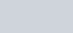This implies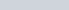Substitute the value of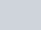, then we get-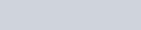In this expression, we can use the geometry of the circular path to find the value of R. See figure below:

In the above figure, you can see that the angles enclosed between pairs of two perpendicular lines are equal. So from this, we have-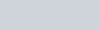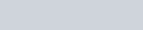Approximate DG to be equal to the length of the magnetic region.

#### Measurement of deflection by the electric field

Again when the magnetic and electric forces got balanced, then switch off the supply of magnetic field and let the electron beam to be deviated only due to the electric field.

When the electron beam moves into the region of the electric field then it experiences an upward force as we discussed earlier. This force acts in upwards y-direction and imparts acceleration to the particle in the y-direction. However, the particle is yet to moves with the same velocity in the x-direction. As a result, the trajectory of motion is parabolic.fig. 8, deflection of the charged particle in an electric field, source: cnx.org

Let L is the length of the charged plate and y be the deflection inside the plate. Then, time taken by the particle to travel through the plate is-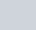And the acceleration of the particle in y-direction is-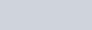The vertical displacement is given as-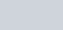After substituting the value of acceleration and time in above expression of displacement, we get-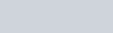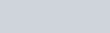Substitute the value ofin above equation, we get-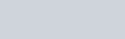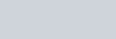This implies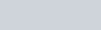From the above diagram, after measuring the GI and HI, we can find the value of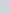and can easily give the required y.

So this is all about the motion of the charged particles in a combined electric and magnetic fields. Stay tuned with Laws Of Nature for more useful and interesting content.